# Concatenate

Use the CONCATENATE function in Excel to concatenate (join) strings. Instead of using CONCATENATE, simply use the & operator.

1. The CONCATENATE function below concatenates the string in cell A1 and the string in cell B1.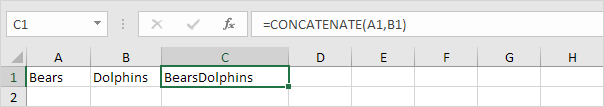2. Use the & operator to produce the exact same result.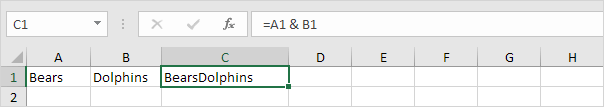3. The CONCATENATE function below concatenates the string in cell A1, the string " and " (enclose text in double quotation marks) and the string in cell B1.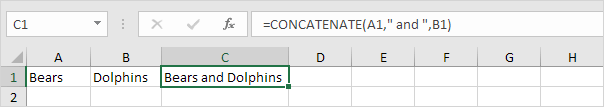4. Use the & operator to produce the exact same result.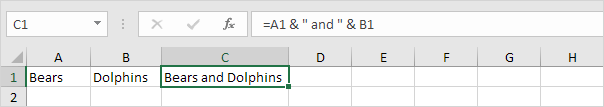5. The CONCATENATE function below concatenates the string in cell A1, a space and the string in cell B1.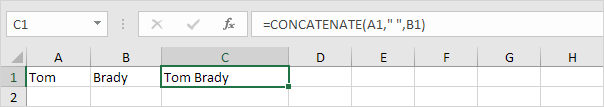6. Use the & operator to produce the exact same result.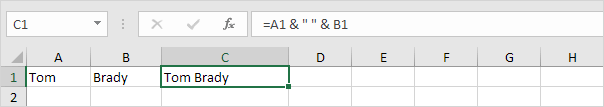The CONCAT function in Excel 2016 or later produces the exact same result as the CONCATENATE function. Simply replace CONCATENATE with CONCAT in the formulas shown above.

7. The CONCAT function can also join a range of strings. If you don't need a delimiter (space, comma, dash, etc.) this can be useful.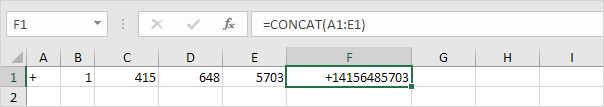The TEXTJOIN function in Excel 2016 or later joins a range of strings using a delimiter (first argument).

8. First, take a look at all the extra spaces in column E below when you drag the CONCATENATE function in cell E2 down to cell E11.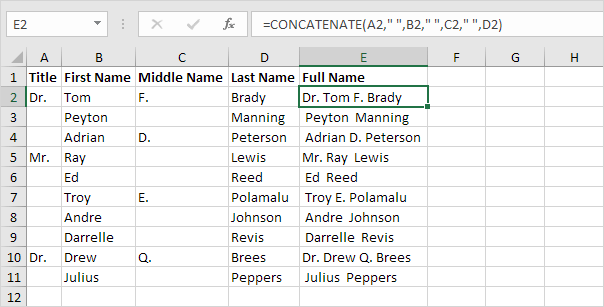9. The beauty of the TEXTJOIN function is that it can ignore empty cells (if the second argument is set to TRUE).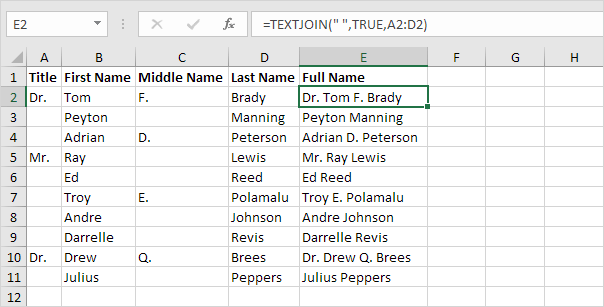10. When using the CONCATENATE function, use the TEXT function to format numbers.Note: use 0 to display the nearest integer value. Use 0.0 for one decimal place. Use 0.00 for two decimal places, etc. Visit our page about the TEXT function for many more examples.

11. Use CHAR(10) to insert a line break.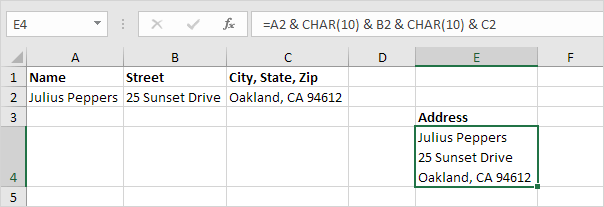Note: don't forget to enable text wrapping. On the Home tab, in the Alignment group, click Wrap Text.

12. Let's take a look at one more cool example that uses the TEXTJOIN function. First, the IF function below finds all members of Team 1.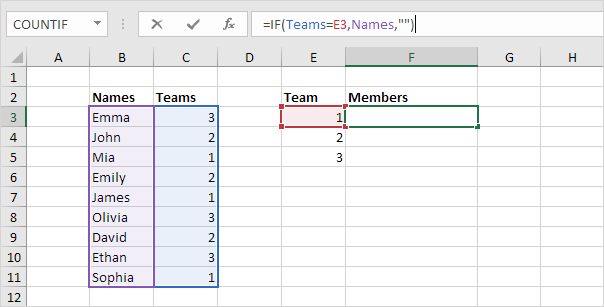Explanation: the IF function checks each cell in the named range Teams. If equal to the value in cell E3, it returns the name from the named range Names, else it returns an empty string (two double quotes with nothing in between).

13. The IF function returns an array constant stored in Excel's memory. Select the formula in the formula bar and press F9 to see this array constant.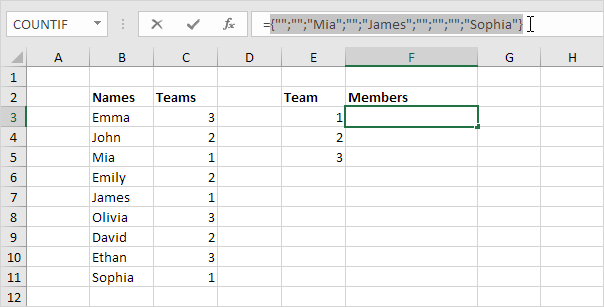14. Add the powerful TEXTJOIN function to concatenate these names. Use a comma and space as the delimiter (first argument) and set the second argument to TRUE to ignore the empty strings. Finish an array formula by pressing CTRL + SHIFT + ENTER.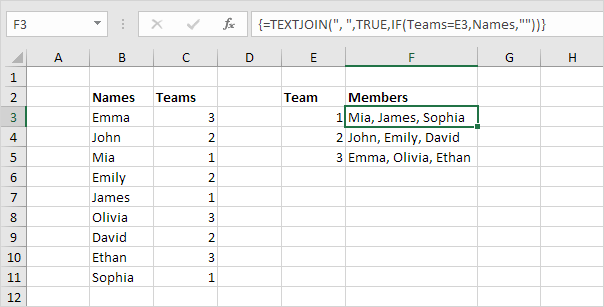Note: Excel adds the curly braces {}. Mia, James and Sophia are in Team 1. Double click the lower right corner of cell F3 to quickly copy this formula to the other cells.